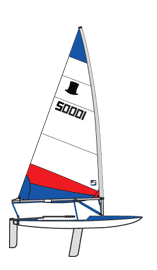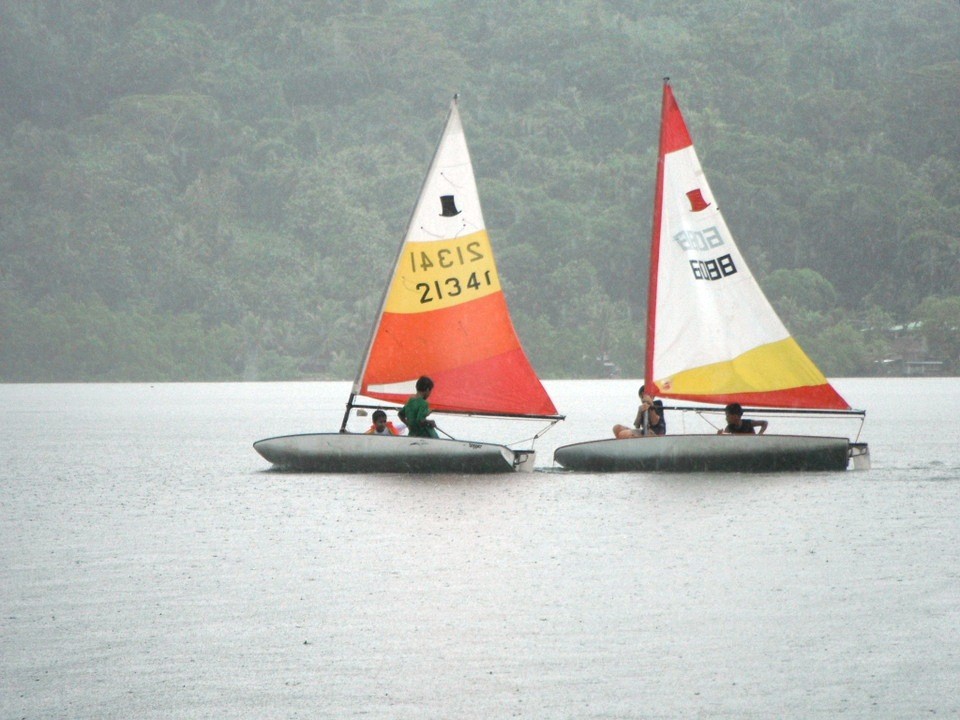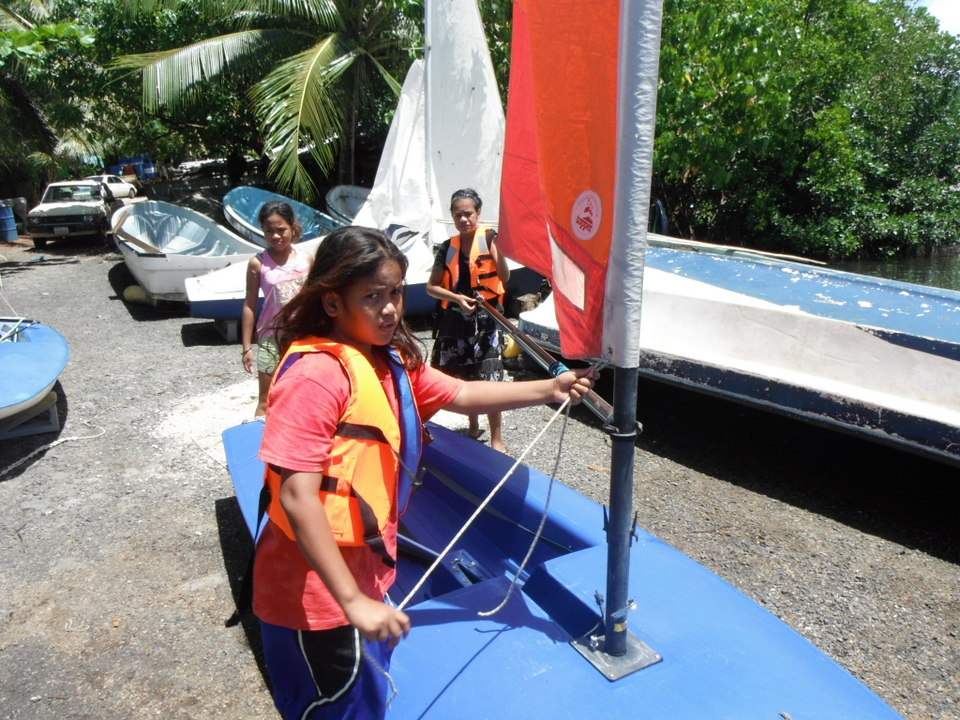Sailing ♒ Name:

Table one
SailboatLength (m)
Optimist2.34
Bug2.60
Taz2.95
Topper3.40
Laser Pico3.50
Vibe3.80
Topaz3.86
Funboat3.90
Magno3.94
Laser4.20
Sunfish4.24
Laser 20004.44

The length of several small sailboats are listed in table one. Use the lengths to answer the following questions.

Data sheet

Part I: Basic Statistics

1. __________ What is the level of measurement for the data in table one?
2. n = __________ Calculate the sample size n.
3. __________ Calculate the minimum.
4. __________ Calculate the maximum.
5. __________ Calculate the range.
6. __________ Calculate the midrange.
7. __________ Calculate the mode.
8. __________ Calculate the median.
9. x = __________ Calculate the sample mean x.
10. sx = __________ Calculate the sample standard deviation sx.
11. __________ Calculate the coefficient of variation.
12. _________ Determine the class width. Use 3 classes (bins or intervals).
13. Fill in the following frequency table:
Classes (x)Frequency fRel Freq p(x)
Sums:
14. Sketch a histogram of the frequency data to the right of the table.
15. __________________ What is the shape of the distribution?
16. __________ Using the sample mean and standard deviation from table one calculate the z-score for a hull with a length of 4.44 meters.
17. __________ Is the z-score for a 4.44 meter hull ordinary or extraordinary?
18. SE = _________ Calculate the standard error of the sample mean x
19. tcritical = _________ Calculate tcritical for a confidence level c of 95%
20. E = _________ Calculate the margin of error E for the sample mean x.
21. Write out the 95% confidence interval for the population mean μ
p(_____________ < μ < ___________) = 0.95

Run a hypothesis test for whether the data in table one could have a population mean hull length of 4 meters. at an alpha α of 0.05.
H0: μ = 4
H1: μ ≠ 4
22. tcritical = _________ Calculate tcritical at α = 0.05.
23. t = _________ Calculate the t-statistic t.
24. p-value = _________ Calculate the p-value.
25. max c = _________ Calculate the maximum confidence that the data in table one has a population mean hull length of 4 meters.
26. _____________________ At an alpha α of 5%, would you fail to reject |or| reject the null hypothesis?

Part II: Hypothesis Testing using the t-test

Topper sailboats are manufactured with a transom mounted mainsheet. The Topper can be converted to a center mounted mainsheet, a system often used in larger racing boats. The hypothesis that a Topper with a transom mounted mainsheet is faster than a Topper with a center mounted mainsheet was tested. Note that the mainsheet is a line which attaches to the boom of the mainsail.

Ten topper sailboats were divided into two groups of five each, one group with transom mounted mainsheets and one group with centre mounted mainsheets. The data is race time in seconds for a single race. Lower times are faster.

The data is NOT paired data, the samples are independent.Table two
Topper mainsheet trials
CenterTransom
12841200
12741253
13471229
12891244
13651279
27. _________ Calculate the sample mean center mounted mainsheet system time
28. _________ Calculate the sample mean transom mounted mainsheet system time
29. _________ Are the sample means for the two samples arithmetically different?
30. __________________ What is the p-value? Use the TTEST function with two tails to determine the p-value for these two independent samples.
31. __________________ Is the difference in the means statistically significant at an alpha α = 0.05?
32. __________________ Would we fail to reject | or | reject a null hypothesis of no difference in the sample means?
33. _____________ What is the maximum level of confidence we can have that the difference is statistically significant?Part III: Linear Regression (best fit or least squares line)
Table three
Length (m)Hull speed (kph)
2.356.90
2.957.73
3.408.30
3.868.84
3.948.93
3.808.77
4.209.22
3.508.42
2.607.26
4.249.27
3.908.89
4.449.48

The data in the table provides the estimated hull speed for a sailboat based on the hull length. The first column is the hull length in meters. The second column is the estimated hull speed in kilometers per hour.
34. _________ Calculate the slope of the linear regression (best fit line).
35. _________ Calculate the y-intercept of the linear regression (best fit line).
36. _________ Is the relation positive, negative, or neutral?
37. _________ Calculate the linear correlation coefficient r for the data.
38. ______________ Is the correlation none, weak/low, moderate, strong/high, or perfect?
39. ______________ Determine the coefficient of determination.
40. ______________ What percent variation in length "explains" the variation in hull speed?
41. ___________ Use the slope and intercept above to predict the hull speed for a 3.5 meter hull length.
42. ___________ Use the slope and intercept above to predict the hull length needed to for a hull speed of 8 kilometers per hour.

n 12
min 2.34
max 4.44
range 2.1
midrange 3.39
mode #VALUE!
median 3.83
mean 3.6
stdev 0.66
cv 0.18
classes 3
width 0.7
cul f rf
3.04 3 0.25
3.74 2 0.17
4.44 7 0.58
5.14 0 0
5.84 0 0
6.54 0 0
7.24 0 0
12

SE0.19
tc 2.2
E0.42
lower 3.18
upper 4.02
mean 3.6
pop mean4
t-2.1
p-value0.06

Part II
Center Transom
n 5 5
mean 1311.8 1241
stdev 41.2 29.25
ttest 0.01591

y = 1.23331x + 4.062

count 12
slope:  1.23
intercept:  4.06
correl:  0.99906
coef det 0.99812
y given x 8.38
x given y 3.19
ttest:  72.83
p-value:  0.0000000 Probably have to omit this due to values.
max c 1.0000000